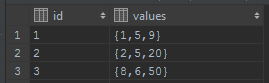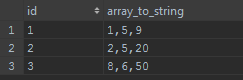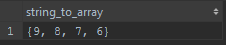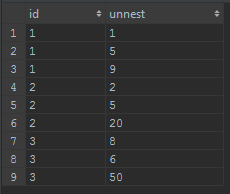# PostgreSQL and it's Array data type

posted on 07 May 2016 | PostgreSQL

Switching between PostgreSQL and SQL Server, I've only ever really used the features of SQL Server in PostgreSQL.

Lately after discovering Marten, I've been exploring features of PostgreSQL.

Array data types, are the new awesome (today as of writing this, for me)

## Defining the table

Using a really boring example, of a table, with an id, and an array of values.

``````drop table if exists test_arrays;

create table test_arrays (
id int,
values int[]
);
``````

Above you can see the values is declared as an array type by adding `[]` to the end of `int`, this declares it as an int array.

PostgreSQL also supports multi-dimentional arrays by adding more square brackets to the end, for example we could define a multi dimentional array of `text` values by declaring the column as `text[][]` which would make it two dimentional.

It also supports fixed size arrays, by passing an integer to the like `text`, this would make the array length of 5.

I'm not going to discuss fixed length or multidimentional arrays in this post tho.

## Inserting Data

Inserting data is a little strange...

``````insert into test_arrays (id, values) values (1, '{1, 5, 9}');
insert into test_arrays (id, values) values (2, '{2, 5, 20}');
insert into test_arrays (id, values) values (3, '{8, 6, 50}');
``````

Here I'm inserting 3 rows, as you can see the arrays are inserted by passing a string value that contains an array, wrapped in curly brackets.

When inserting string values, they need to be wrapped in double quotes, for example:

``````'{"Banana", "Apple", "Watermelon"}'
``````

This would be a value array of strings for use in `text[]`.

An alternative approach is to use the `array` type passing in the values to the constructor like so:

``````insert into test_arrays (id, values) values (4, array[12, 7, 3]);
``````

or

``````insert into test_arrays (id, values) values (4, array["Banana", "Apple", "Watermelon"]);
``````

I prefer the string approach.

## Filtering data

So there's probably 3 main scenarios I can think of when filtering an array.

Given the data we inserted above

#### Does the array contain the value 5?

``````-- does the column contain 5?
select * from test_arrays where 5 = any(values);
``````

This says, does the value of 5 equal any of the values in the array. It's a little strange when you write it the first time but it does make sense.

This would result in rows 1 and 2 being returned.

#### Does the array contain atleast 1 of the values from another array?

``````-- does the column contain at-least one of
select * from test_arrays where values && array[6, 9];
select * from test_arrays where values && '{6, 9}'::int[];
``````

This uses the && operator which is 'overlap', in other words, does the (left) array contain any of the elements in the (right) array.

This would result in rows 1 and 3 being returned.

I wrote this using both ways of writing the array.

#### Does the array NOT contain any of the values from another array?

Very similar to the contains, only we specify it as `not` before the condition.

``````-- does the column NOT contain at-least one of
select * from test_arrays where not values && array[6, 9];
select * from test_arrays where not values && '{6, 9}'::int[];
``````

This would result in 1 row being returned.

#### Does the array contain all of the values from another array?

``````-- does the column contain all of
select * from test_arrays where values @> array[6, 9];
select * from test_arrays where values @> '{6, 9}'::int[];
``````

This uses the contains operator, so we're saying, given the (left) array of values, does it contain all of the values in the (right) array values.

This would result in row 1 being returned.

## Returning data

If you're selecting out your array, it will be selected as the format that we inserted it as.This makes parsing it a little hard. In C# you would get a string back, and have to strip off the `{ }` and then split on `,`. The good news is there are options to help you return the data a little nicer.

#### array_to_string to remove curly brackets

Using `array_to_string(...)` function, we can return the results as a string without the brackets.

``````-- selecting the array as a string
select id, array_to_string(values, ',') from test_arrays;
``````

This will return the same result as above, without the `{ }`There's also the opposite, `string_to_array`, which will allow you to pass a string in and get an array back.

``````-- returning the array as a string
select string_to_array('9, 8, 7, 6', ',');
``````

This would give you:#### unnest to return an array as rows

This is so awesome, trying to do this in SQL Server is SUCH a pain. PostgreSQL supports `unnest` which will return each value of an array, as a row, it will also duplicate all data from other rows to each row returned for the array.

``````-- return array column as rows
select id, unnest(values) from test_arrays
``````

This results in the following result:## Indexes & Doco

There's a lot of other stuff that I haven't included in this post. The type is Indexable, so you could apply a GIN index against the column for faster searching against `=`, `&&` and `<@` / `>@` operators.

http://www.postgresql.org/docs/9.5/static/gin-builtin-opclasses.html

The PostgreSQL documentation is awesome. Check it out.

http://www.postgresql.org/docs/9.5/static/functions-array.html